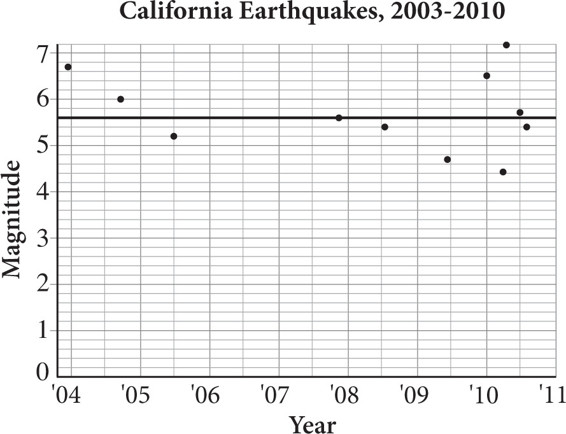# SAT Math Multiple Choice Question 479: Answer and Explanation

### Test Information

Question: 479

14.Earthquakes occur when energy is released from deep inside the earth, causing friction between the tectonic plates of the earth's crust. The magnitude, or intensity, of the earthquake is measured on the Richter scale. The scatterplot above shows the earthquakes experienced by California between December 2003 and December 2010. The line of best fit, which has a slope of 0, is a fairly good indicator in California. Approximately what percent of the earthquakes in California during this time period differed by 1 point or more on the Richter scale from the magnitude predicted by the line of best fit?

• A. 22%
• B. 27%
• C. 33%
• D. 36%

Explanation:

B

Difficulty: Hard

Category: Problem Solving and Data Analysis / Scatterplots

Strategic Advice: Being able to read information from an infographic is an important skill that will earn you points on Test Day.

Getting to the Answer: Examine the graph, including the axis labels and numbering. On the vertical axis, there are 5 parts to each 1 unit, which means each grid-line represents 0.2 points on the Richter scale. So you are looking for points that are 5 or more grid-lines away from the line of best fit. There are 3 points that meet this criterion (one before 2004 and two between 2010 and 2011). Now, count the total number of data points: There are 11, and 3 ÷ 11 = .2727, or about 27 percent.

Note that you could also do the math to find that the magnitude predicted by the line of best fit is 5.6, so you're looking for points with a y-value of 4.6 or lower and 6.6 or greater.